moveit2 The MoveIt Motion Planning Framework for ROS 2.
distance_field::PropagationDistanceField Class Reference

A DistanceField implementation that uses a vector propagation method. Distances propagate outward from occupied cells, or inwards from unoccupied cells if negative distances are to be computed, which is optional. Outward and inward propagation only occur to a desired maximum distance - cells that are more than this maximum distance from the nearest cell will have maximum distance measurements. More...

`#include <propagation_distance_field.h>`

Inheritance diagram for distance_field::PropagationDistanceField: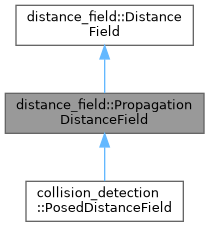[legend]
Collaboration diagram for distance_field::PropagationDistanceField: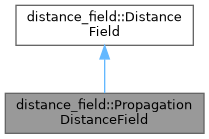[legend]

## Public Member Functions

PropagationDistanceField (double size_x, double size_y, double size_z, double resolution, double origin_x, double origin_y, double origin_z, double max_distance, bool propagate_negative_distances=false)
Constructor that initializes entire distance field to empty - all cells will be assigned maximum distance values. All units are arbitrary but are assumed for documentation purposes to represent meters. More...

PropagationDistanceField (const octomap::OcTree &octree, const octomap::point3d &bbx_min, const octomap::point3d &bbx_max, double max_distance, bool propagate_negative_distances=false)
Constructor based on an OcTree and bounding box information. A distance field will be constructed with dimensions based on the supplied bounding box at the resolution of the OcTree. All octree obstacle cells will be added to the resulting distance field using the DistanceField::addOcTreeToField function. More...

PropagationDistanceField (std::istream &stream, double max_distance, bool propagate_negative_distances=false)
Constructor that takes an istream and reads the contents of a saved distance field, adding all obstacle points and running propagation given the arguments for max_distance and propagate_negative_distances. Calls the function readFromStream. More...

~PropagationDistanceField () override
Empty destructor. More...

void addPointsToField (const EigenSTL::vector_Vector3d &points) override
Add a set of obstacle points to the distance field, updating distance values accordingly. The distance field may already contain obstacle cells. More...

void removePointsFromField (const EigenSTL::vector_Vector3d &points) override
Remove a set of obstacle points from the distance field, updating distance values accordingly. More...

void updatePointsInField (const EigenSTL::vector_Vector3d &old_points, const EigenSTL::vector_Vector3d &new_points) override
This function will remove any obstacle points that are in the old point set but not the new point set, and add any obstacle points that are in the new block set but not the old block set. Any points that are in both sets are left unchanged. For more information see DistanceField::updatePointsInField. More...

void reset () override
Resets the entire distance field to max_distance for positive values and zero for negative values. More...

double getDistance (double x, double y, double z) const override
Get the distance value associated with the cell indicated by the world coordinate. If the cell is invalid, max_distance will be returned. If running without negative distances, all obstacle cells will have zero distance. If running with negative distances, the distance will be between -max_distance and max_distance, with no values having a 0 distance. More...

double getDistance (int x, int y, int z) const override
Get the distance value associated with the cell indicated by the index coordinates. If the cell is invalid, max_distance will be returned. If running without negative distances, all obstacle cells will have zero distance. If running with negative distances, the distance will be between -max_distance and max_distance, with no values having a 0 distance. More...

bool isCellValid (int x, int y, int z) const override
Determines whether or not the cell associated with the supplied indices is valid for this distance field. More...

int getXNumCells () const override
Gets the number of cells along the X axis. More...

int getYNumCells () const override
Gets the number of cells along the Y axis. More...

int getZNumCells () const override
Gets the number of cells along the Z axis. More...

bool gridToWorld (int x, int y, int z, double &world_x, double &world_y, double &world_z) const override
Converts from an set of integer indices to a world location given the origin and resolution parameters. More...

bool worldToGrid (double world_x, double world_y, double world_z, int &x, int &y, int &z) const override
Converts from a world location to a set of integer indices. Should return false if the world location is not valid in the distance field, and should populate the index values in either case. More...

bool writeToStream (std::ostream &stream) const override
Writes the contents of the distance field to the supplied stream. More...

Reads, parameterizes, and populates the distance field based on the supplied stream. More...

double getUninitializedDistance () const override
Gets a distance value for an invalid cell. More...

const PropDistanceFieldVoxelgetCell (int x, int y, int z) const
Gets full cell data given an index. More...

const PropDistanceFieldVoxelgetNearestCell (int x, int y, int z, double &dist, Eigen::Vector3i &pos) const
Gets nearest surface cell and returns distance to it. More...

int getMaximumDistanceSquared () const
Gets the maximum distance squared value. More...Public Member Functions inherited from distance_field::DistanceField
DistanceField (double size_x, double size_y, double size_z, double resolution, double origin_x, double origin_y, double origin_z)
Constructor, where units are arbitrary but are assumed to be meters. More...

virtual ~DistanceField ()

bool getShapePoints (const shapes::Shape *shape, const Eigen::Isometry3d &pose, EigenSTL::vector_Vector3d *points)
Get the points associated with a shape. This is mainly used when the external application needs to cache points. More...

void addShapeToField (const shapes::Shape *shape, const Eigen::Isometry3d &pose)
Adds the set of points corresponding to the shape at the given pose as obstacle points into the distance field. If the shape is an OcTree, the pose information is ignored and the OcTree is passed to the addOcTreeToField function. More...

Adds an octree to the distance field. Cells that are occupied in the octree that lie within the voxel grid are added to the distance field. The octree can represent either a larger or smaller volume than the distance field. If the resolution of the octree is less than or equal to the resolution of the distance field then the center of each leaf cell of the octree will be added to the distance field. If the resolution of the octree is greater than a 3D volume of the correct resolution will be added for each occupied leaf node. More...

void moveShapeInField (const shapes::Shape *shape, const Eigen::Isometry3d &old_pose, const Eigen::Isometry3d &new_pose)
Moves the shape in the distance field from the old pose to the new pose, removing points that are no longer obstacle points, and adding points that are now obstacle points at the new pose. This function will discretize both shapes, and call the updatePointsInField function on the old and new point sets. More...

void removeShapeFromField (const shapes::Shape *shape, const Eigen::Isometry3d &pose)
All points corresponding to the shape are removed from the distance field. More...

Gets not only the distance to the nearest cell but the gradient direction. The gradient is computed as a function of the distances of near-by cells. An uninitialized distance is returned if the cell is not valid for gradient production purposes. The gradient is pointing out of the obstacle - thus to recover the closest obstacle point, the normalized gradient value is multiplied by the distance and subtracted from the cell's location, as shown below. More...

void getIsoSurfaceMarkers (double min_distance, double max_distance, const std::string &frame_id, const rclcpp::Time &stamp, visualization_msgs::msg::Marker &marker) const
Get an iso-surface for visualization in rviz. The iso-surface shows every cell that has a distance in a given range in the distance field. The cells are added as a visualization_msgs::msg::Marker::CUBE_LIST in the namespace "distance_field". More...

Populates the supplied marker array with a series of arrows representing gradients of cells that are within the supplied range in terms of distance. The markers will be visualization_msgs::msg::Marker::ARROW in the namespace "distance_field_gradient". More...

void getPlaneMarkers (PlaneVisualizationType type, double length, double width, double height, const Eigen::Vector3d &origin, const std::string &frame_id, const rclcpp::Time &stamp, visualization_msgs::msg::Marker &marker) const
Populates a marker with a slice of the distance field in a particular plane. All cells in the plane will be added to the field, with colors associated with their distance. More...

void getProjectionPlanes (const std::string &frame_id, const rclcpp::Time &stamp, double max_distance, visualization_msgs::msg::Marker &marker) const
A function that populates the marker with three planes - one each along the XY, XZ, and YZ axes. For each of the planes, any column on that plane will be marked according to the minimum distance along that column. More...

double getSizeX () const
Gets the distance field size along the X dimension in meters. More...

double getSizeY () const
Gets the distance field size along the Y dimension in meters. More...

double getSizeZ () const
Gets the distance field size along the Z dimension in meters. More...

double getOriginX () const
Gets the origin (minimum value) along the X dimension. More...

double getOriginY () const
Gets the origin (minimum value) along the Y dimension. More...

double getOriginZ () const
Gets the origin (minimum value) along the Z dimension. More...

double getResolution () const
Gets the resolution of the distance field in meters. More...Protected Member Functions inherited from distance_field::DistanceField
void getOcTreePoints (const octomap::OcTree *octree, EigenSTL::vector_Vector3d *points)
Get the points associated with an octree. More...

void setPoint (int xCell, int yCell, int zCell, double dist, geometry_msgs::msg::Point &point, std_msgs::msg::ColorRGBA &color, double max_distance) const
Helper function that sets the point value and color given the distance. More...Protected Attributes inherited from distance_field::DistanceField
double size_x_
X size of the distance field. More...

double size_y_
Y size of the distance field. More...

double size_z_
Z size of the distance field. More...

double origin_x_
X origin of the distance field. More...

double origin_y_
Y origin of the distance field. More...

double origin_z_
Z origin of the distance field. More...

double resolution_
Resolution of the distance field. More...

int inv_twice_resolution_
Computed value 1.0/(2.0*resolution_) More...

## Detailed Description

A DistanceField implementation that uses a vector propagation method. Distances propagate outward from occupied cells, or inwards from unoccupied cells if negative distances are to be computed, which is optional. Outward and inward propagation only occur to a desired maximum distance - cells that are more than this maximum distance from the nearest cell will have maximum distance measurements.

This class uses a VoxelGrid to hold all data. One important decision that must be made on construction is whether or not to create a signed version of the distance field. If the distance field is unsigned, it means that the minimum obstacle distance is 0, a value that will be assigned to all obstacle cells. Gradient queries for obstacle cells will not give useful information, as the gradient at an obstacle cell will point to the cell itself. If this behavior is acceptable, then the performance of this mode will be more efficient, as no propagation will occur for obstacle cells. The other option is to calculate signed distances. In this case, negative distances up to the maximum distance are calculated for obstacle volumes. This distance encodes the distance of an obstacle cell to the nearest unoccupied obstacle voxel. Furthmore, gradients pointing out of the volume will be produced. Depending on the data, calculating this data can significantly impact the time it takes to add and remove obstacle cells.

Definition at line 144 of file propagation_distance_field.h.

## ◆ PropagationDistanceField() [1/3]

 distance_field::PropagationDistanceField::PropagationDistanceField ( double size_x, double size_y, double size_z, double resolution, double origin_x, double origin_y, double origin_z, double max_distance, bool propagate_negative_distances = `false` )

Constructor that initializes entire distance field to empty - all cells will be assigned maximum distance values. All units are arbitrary but are assumed for documentation purposes to represent meters.

Parameters
 [in] size_x The X dimension in meters of the volume to represent [in] size_y The Y dimension in meters of the volume to represent [in] size_z The Z dimension in meters of the volume to represent [in] resolution The resolution in meters of the volume [in] origin_x The minimum X point of the volume [in] origin_y The minimum Y point of the volume [in] origin_z The minimum Z point of the volume [in] max_distance The maximum distance to which to propagate distance values. Cells that are greater than this distance will be assigned the maximum distance value. [in] propagate_negative_distances Whether or not to propagate negative distances. If false, no propagation occurs, and all obstacle cells will be assigned zero distance. See the PropagationDistanceField description for more information on the implications of this.

Definition at line 50 of file propagation_distance_field.cpp.

## ◆ PropagationDistanceField() [2/3]

 distance_field::PropagationDistanceField::PropagationDistanceField ( const octomap::OcTree & octree, const octomap::point3d & bbx_min, const octomap::point3d & bbx_max, double max_distance, bool propagate_negative_distances = `false` )

Constructor based on an OcTree and bounding box information. A distance field will be constructed with dimensions based on the supplied bounding box at the resolution of the OcTree. All octree obstacle cells will be added to the resulting distance field using the DistanceField::addOcTreeToField function.

Parameters
 [in] octree The OcTree from which to construct the distance field [in] bbx_min The minimum world coordinates of the bounding box [in] bbx_max The maximum world coordinates of the bounding box [in] max_distance The maximum distance to which to propagate distance values. Cells that are greater than this distance will be assigned the maximum distance value. [in] propagate_negative_distances Whether or not to propagate negative distances. If false, no propagation occurs, and all obstacle cells will be assigned zero distance. See the PropagationDistanceField description for more information on the implications of this.

Definition at line 60 of file propagation_distance_field.cpp.

Here is the call graph for this function: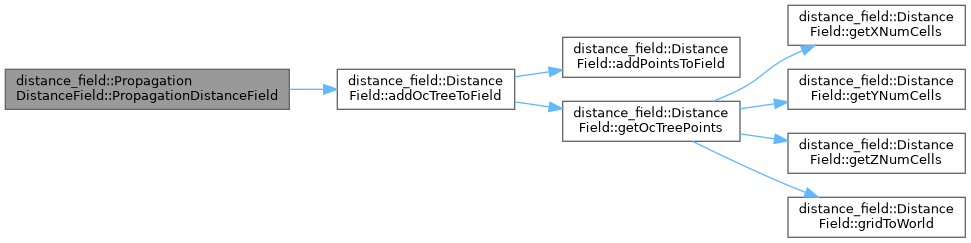## ◆ PropagationDistanceField() [3/3]

 distance_field::PropagationDistanceField::PropagationDistanceField ( std::istream & stream, double max_distance, bool propagate_negative_distances = `false` )

Constructor that takes an istream and reads the contents of a saved distance field, adding all obstacle points and running propagation given the arguments for max_distance and propagate_negative_distances. Calls the function readFromStream.

Parameters
 [in] stream The stream from which to read the data [in] max_distance The maximum distance to which to propagate distance values. Cells that are greater than this distance will be assigned the maximum distance value. [in] propagate_negative_distances Whether or not to propagate negative distances. If false, no propagation occurs, and all obstacle cells will be assigned zero distance. See the PropagationDistanceField description for more information on the implications of this.
Returns

Definition at line 73 of file propagation_distance_field.cpp.

Here is the call graph for this function: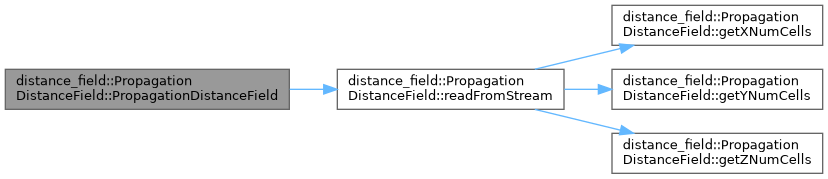## ◆ ~PropagationDistanceField()

 distance_field::PropagationDistanceField::~PropagationDistanceField ( )
inlineoverride

Empty destructor.

Definition at line 229 of file propagation_distance_field.h.

## Member Function Documentation

 void distance_field::PropagationDistanceField::addPointsToField ( const EigenSTL::vector_Vector3d & points )
overridevirtual

Add a set of obstacle points to the distance field, updating distance values accordingly. The distance field may already contain obstacle cells.

The function first checks that each location represents a valid point - only valid points will be added. It takes the vector of valid points and performs positive propagation on them. If the class has been set up to propagate negative distance, those will also be propagated.

Parameters
 [in] points The set of obstacle points to add

Implements distance_field::DistanceField.

Definition at line 171 of file propagation_distance_field.cpp.

Here is the call graph for this function: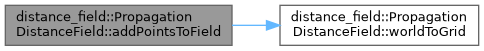## ◆ getCell()

 const PropDistanceFieldVoxel& distance_field::PropagationDistanceField::getCell ( int x, int y, int z ) const
inline

Gets full cell data given an index.

x,y,z MUST be valid or data corruption (SEGFAULTS) will occur.

Parameters
 [in] x The integer X location [in] y The integer Y location [in] z The integer Z location
Returns
The data in the indicated cell.

Definition at line 395 of file propagation_distance_field.h.

Here is the caller graph for this function:## ◆ getDistance() [1/2]

 double distance_field::PropagationDistanceField::getDistance ( double x, double y, double z ) const
overridevirtual

Get the distance value associated with the cell indicated by the world coordinate. If the cell is invalid, max_distance will be returned. If running without negative distances, all obstacle cells will have zero distance. If running with negative distances, the distance will be between -max_distance and max_distance, with no values having a 0 distance.

Parameters
 [in] x The X location of the cell [in] y The X location of the cell [in] z The X location of the cell
Returns
The distance value

Implements distance_field::DistanceField.

Definition at line 588 of file propagation_distance_field.cpp.

Here is the caller graph for this function: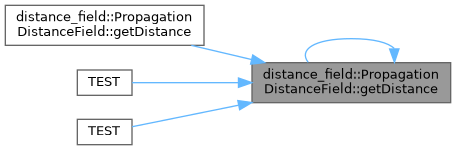## ◆ getDistance() [2/2]

 double distance_field::PropagationDistanceField::getDistance ( int x, int y, int z ) const
overridevirtual

Get the distance value associated with the cell indicated by the index coordinates. If the cell is invalid, max_distance will be returned. If running without negative distances, all obstacle cells will have zero distance. If running with negative distances, the distance will be between -max_distance and max_distance, with no values having a 0 distance.

Parameters
 [in] x The integer X location [in] y The integer Y location [in] z The integer Z location
Returns
The distance value for the cell

Implements distance_field::DistanceField.

Definition at line 593 of file propagation_distance_field.cpp.

Here is the call graph for this function: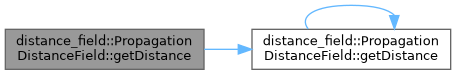## ◆ getMaximumDistanceSquared()

 int distance_field::PropagationDistanceField::getMaximumDistanceSquared ( ) const
inline

Gets the maximum distance squared value.

Produced by taking the ceiling of the maximum distance divided by the resolution, and then squaring that value.

Returns
The maximum distance squared.

Definition at line 450 of file propagation_distance_field.h.

## ◆ getNearestCell()

 const PropDistanceFieldVoxel* distance_field::PropagationDistanceField::getNearestCell ( int x, int y, int z, double & dist, Eigen::Vector3i & pos ) const
inline

Gets nearest surface cell and returns distance to it.

x,y,z MUST be valid or data corruption (SEGFAULTS) will occur.

Parameters
 [in] x The integer X location of the starting cell [in] y The integer Y location of the starting cell [in] z The integer Z location of the starting cell [out] dist if starting cell is inside, the negative distance to the nearest outside cell if starting cell is outside, the positive distance to the nearest inside cell if nearby cell is unknown, zero [out] pos the position of the nearest cell
Returns
If starting cell is inside, the nearest outside cell If starting cell is outside, the nearst inside cell If nearest cell is unknown, return nullptr

Definition at line 418 of file propagation_distance_field.h.

## ◆ getUninitializedDistance()

 double distance_field::PropagationDistanceField::getUninitializedDistance ( ) const
inlineoverridevirtual

Gets a distance value for an invalid cell.

Returns
The distance associated with an uninitialized cell

Implements distance_field::DistanceField.

Definition at line 379 of file propagation_distance_field.h.

## ◆ getXNumCells()

 int distance_field::PropagationDistanceField::getXNumCells ( ) const
overridevirtual

Gets the number of cells along the X axis.

Returns
The number of cells along the X axis

Implements distance_field::DistanceField.

Definition at line 603 of file propagation_distance_field.cpp.

Here is the caller graph for this function: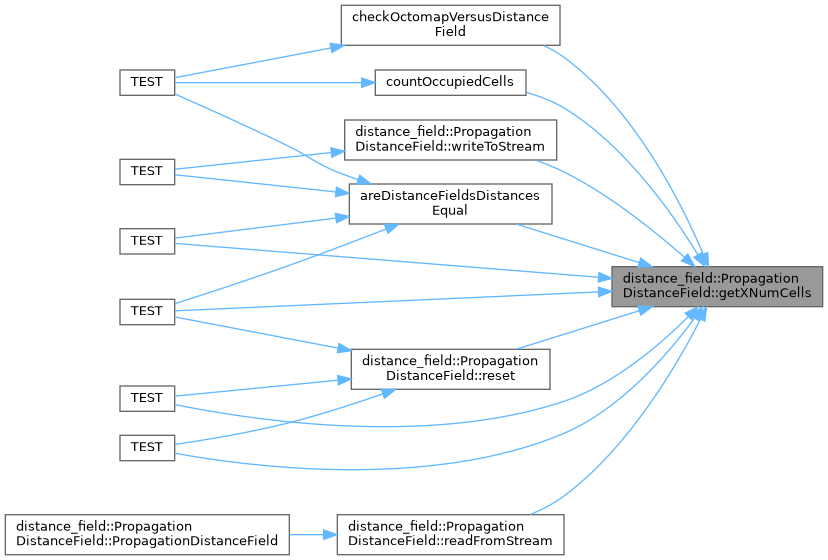## ◆ getYNumCells()

 int distance_field::PropagationDistanceField::getYNumCells ( ) const
overridevirtual

Gets the number of cells along the Y axis.

Returns
The number of cells along the Y axis

Implements distance_field::DistanceField.

Definition at line 608 of file propagation_distance_field.cpp.

Here is the caller graph for this function: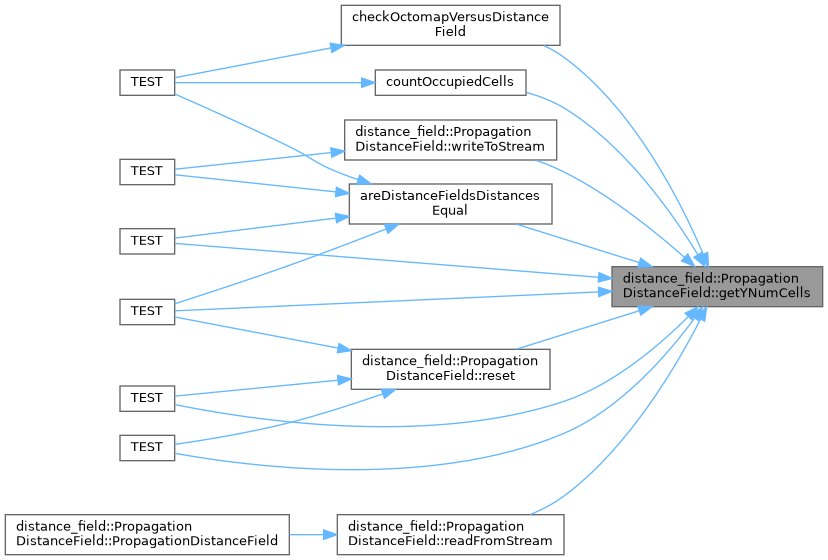## ◆ getZNumCells()

 int distance_field::PropagationDistanceField::getZNumCells ( ) const
overridevirtual

Gets the number of cells along the Z axis.

Returns
The number of cells along the Z axis

Implements distance_field::DistanceField.

Definition at line 613 of file propagation_distance_field.cpp.

Here is the caller graph for this function: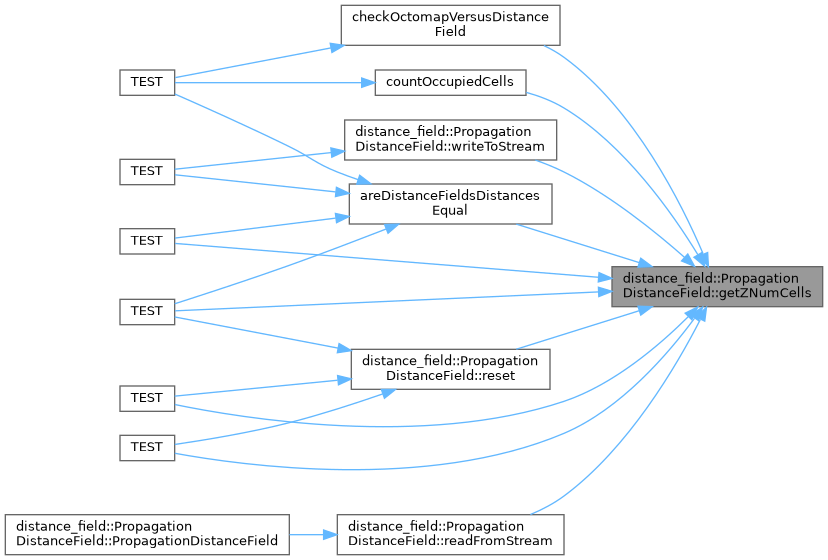## ◆ gridToWorld()

 bool distance_field::PropagationDistanceField::gridToWorld ( int x, int y, int z, double & world_x, double & world_y, double & world_z ) const
overridevirtual

Converts from an set of integer indices to a world location given the origin and resolution parameters.

Parameters
 [in] x The integer X location [in] y The integer Y location [in] z The integer Z location [out] world_x The computed world X location [out] world_y The computed world X location [out] world_z The computed world X location
Returns
Whether or not the transformation is successful. An implementation may or may not choose to return false if the indicated cell is not valid for this distance field.

Implements distance_field::DistanceField.

Definition at line 618 of file propagation_distance_field.cpp.

Here is the caller graph for this function: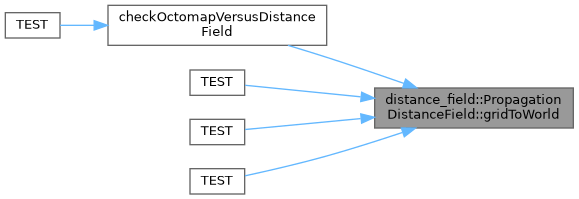## ◆ isCellValid()

 bool distance_field::PropagationDistanceField::isCellValid ( int x, int y, int z ) const
overridevirtual

Determines whether or not the cell associated with the supplied indices is valid for this distance field.

Parameters
 [in] x The X index of the cell [in] y The Y index of the cell [in] z The Z index of the cell
Returns
True if the cell is valid, otherwise false.

Implements distance_field::DistanceField.

Definition at line 598 of file propagation_distance_field.cpp.

 bool distance_field::PropagationDistanceField::readFromStream ( std::istream & stream )
overridevirtual

Reads, parameterizes, and populates the distance field based on the supplied stream.

This function assumes that the file begins with ASCII data, and that the binary data has been written in bit formulation and compressed using Zlib. The function will reinitialize all data members based on the data in the file, using preset values for max_distance_ and propagate_negative_distances_. All occupied cells will be added to the distance field.

Parameters
 [in] stream The stream from which to read
Returns
True if reading, parameterizing, and populating the distance field is successful; otherwise False.

Implements distance_field::DistanceField.

Definition at line 669 of file propagation_distance_field.cpp.

Here is the call graph for this function: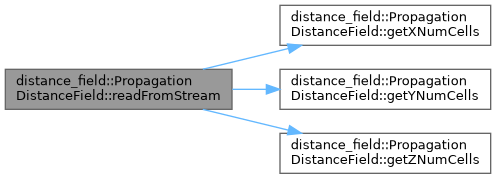Here is the caller graph for this function: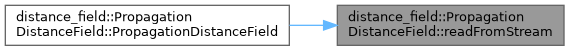## ◆ removePointsFromField()

 void distance_field::PropagationDistanceField::removePointsFromField ( const EigenSTL::vector_Vector3d & points )
overridevirtual

Remove a set of obstacle points from the distance field, updating distance values accordingly.

This function is relatively less efficient than adding points to the field in terms of positive distances - adding a given number of points will be less comptationally expensive than removing the same number of points. This is due to the nature of the propagation algorithm - when removing sets of cells, we must search outward from the freed cells and then propagate inward. Negative distances can be propagated more efficiently, as propagation can occur outward from newly freed cells without requiring a search step. If the set of occupied points that remain after removal is small it may be more efficient to call reset and then to add the remaining points rather than removing a set of points.

Parameters
 [in] points The set of obstacle points that will be set as free

Implements distance_field::DistanceField.

Definition at line 192 of file propagation_distance_field.cpp.

Here is the call graph for this function: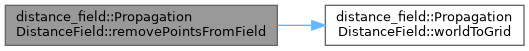## ◆ reset()

 void distance_field::PropagationDistanceField::reset ( )
overridevirtual

Resets the entire distance field to max_distance for positive values and zero for negative values.

Implements distance_field::DistanceField.

Definition at line 500 of file propagation_distance_field.cpp.

Here is the call graph for this function: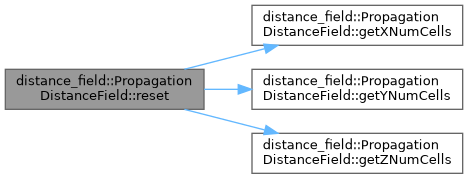## ◆ updatePointsInField()

 void distance_field::PropagationDistanceField::updatePointsInField ( const EigenSTL::vector_Vector3d & old_points, const EigenSTL::vector_Vector3d & new_points )
overridevirtual

This function will remove any obstacle points that are in the old point set but not the new point set, and add any obstacle points that are in the new block set but not the old block set. Any points that are in both sets are left unchanged. For more information see DistanceField::updatePointsInField.

The implementation of this function finds the set of points that are in the old_points and not the new_points, and the in the new_points and not the old_points using std::set_difference. It then calls a removal function on the former set, and an addition function on the latter set.

If there is no overlap between the old_points and the new_points it is more efficient to first call removePointsFromField on the old_points and then addPointsToField on the new points - this does not require computing set differences.

Parameters
 [in] old_points The set of points that all should be obstacle cells in the distance field [in] new_points The set of points, all of which are intended to be obstacle points in the distance field

Implements distance_field::DistanceField.

Definition at line 124 of file propagation_distance_field.cpp.

Here is the call graph for this function: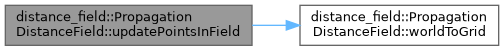## ◆ worldToGrid()

 bool distance_field::PropagationDistanceField::worldToGrid ( double world_x, double world_y, double world_z, int & x, int & y, int & z ) const
overridevirtual

Converts from a world location to a set of integer indices. Should return false if the world location is not valid in the distance field, and should populate the index values in either case.

Parameters
 [in] world_x The world X location [in] world_y The world Y location [in] world_z The world Z location [out] x The computed integer X location [out] y The computed integer X location [out] z The computed integer X location
Returns
True if all the world values result in integer indices that pass a validity check; otherwise False.

Implements distance_field::DistanceField.

Definition at line 624 of file propagation_distance_field.cpp.

Here is the caller graph for this function: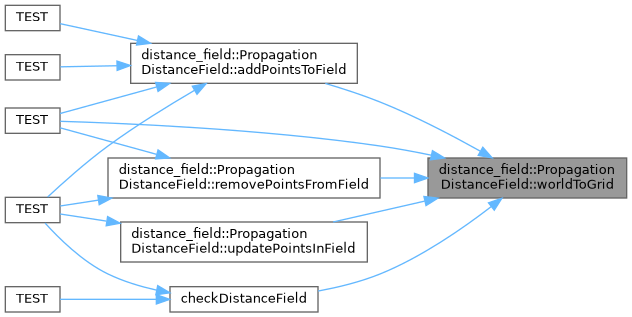## ◆ writeToStream()

 bool distance_field::PropagationDistanceField::writeToStream ( std::ostream & stream ) const
overridevirtual

Writes the contents of the distance field to the supplied stream.

This function writes the resolution, size, and origin parameters to the file in ASCII. It then writes the occupancy data only in bit form (with values or 1 representing occupancy, and 0 representing empty space). It further runs Zlib compression on the binary data before actually writing to disk. The max_distance and propagate_negative_distances values are not written to file, and the distances themselves will need to be recreated on load.

Parameters
 [out] stream The stream to which to write the distance field contents.
Returns
True

Implements distance_field::DistanceField.

Definition at line 629 of file propagation_distance_field.cpp.

Here is the call graph for this function: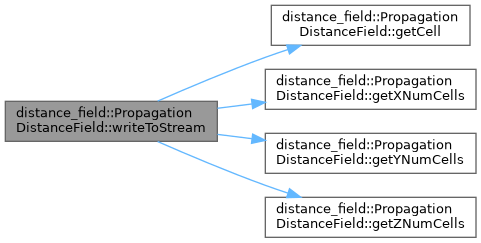The documentation for this class was generated from the following files: